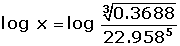Chapters

A logarithm, positive or negative, corresponds to every positive number.The antilogarithm is the number for which a given logarithm stands. For example, if log x equals y, then x is the antilogarithm of y.To find the antilog of a number in a given base, raise the base to the number result.If the logarithm is known, a calculator can be used to find the antilog by pressing the 10x key. This is usually the second function of the log key.

log x = 2.4572

x = 102.4572 = 286.55

### Cologarithm

The cologarithm of a number is the logarithm of its inverse, that is to say, the cologarithm of a number is the opposite of its logarithm.colog 200 = -log 200 = -2.3010

## Examples

Using logarithms, calcaute the value of x.

123Did you like the article?(1 votes, average: 5.00 out of 5)Loading...

Emma

I am passionate about travelling and currently live and work in Paris. I like to spend my time reading, gardening, running, learning languages and exploring new places.

Did you like
this resource?

Bravo!